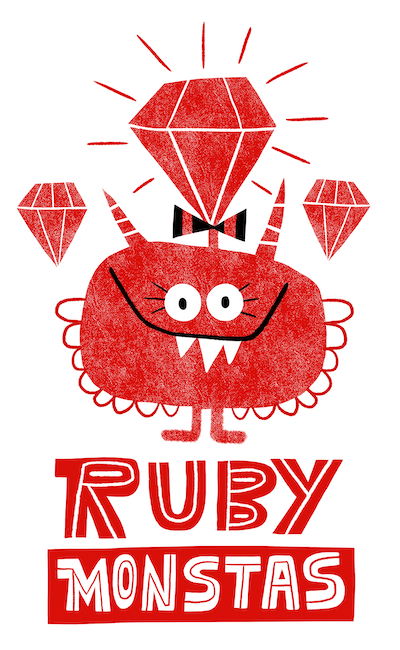# Ruby Monstas

Variables## Variables

IRB: As soon as you hit Enter and the Ruby command has finished executing, the result is forgotten

We should be able to use the result of one line in the next

Enter variables!

## The drawer metaphorYou can think of variables like drawers in an apothecary

The drawers each have a label on them and they contain a specific thing at any time (or nothing)

## Assigning a variable

``my_string = "Hello world"``
``a_number = 5 + 2``

## The drawer metaphor: Assignment## Syntax

``my_string = "Hello world"``
``a_number = 5 + 2``
Note:
`=`
is called the assignment operator
Note:
`=`
differs from how it's used in mathematics
Note: We're defining the variable and initializing it with a value
Note: Syntax is
`variable_name = expression`

## Example expressions

``5``
``"Hello world"``
``a_number.odd?``
``my_string.include?("Hello")``
``8 * 6``
``(a_number / 5.0) + 42``

## Expressions

An expression is any piece of Ruby code that returns a value

Any expression can be assigned to a variable!

``b_number = (a_number / 5.0) + 42``

## Reassignment

You can give existing variables a new value by reassigning

``````a_number = 5 + 2
a_number = 42``````

Note: Note syntax is the same as for assignment

Note: The old value of the variable is lost by doing this

## Reassignment

You can also use the variable in an expression when reassigning it

``````a_number = 5 + 2
a_number = a_number + 3``````

Note: Here's where it definitely stops making sense mathematically ;-)

## Variable types

A variable always has a specific type

For example String or Integer

Whenever Ruby executes a method on a variable, it first checks the type!

``````a_number = 5 + 2
a_number.length``````
`NoMethodError (undefined method `length' for 7:Integer)`

## Variable names

Which of these are good variable names?

```mynewvariable
user_name
date_of_birth
5_things
AnotherVariableName
list_of_favourite_drinks```

## Variable names

These characters are allowed:

• lower-case letters (
`a - z`
)
• digits (
`0 - 9`
)
• underscore (
`_`
)
Variable names can't contain spaces (use
`_`